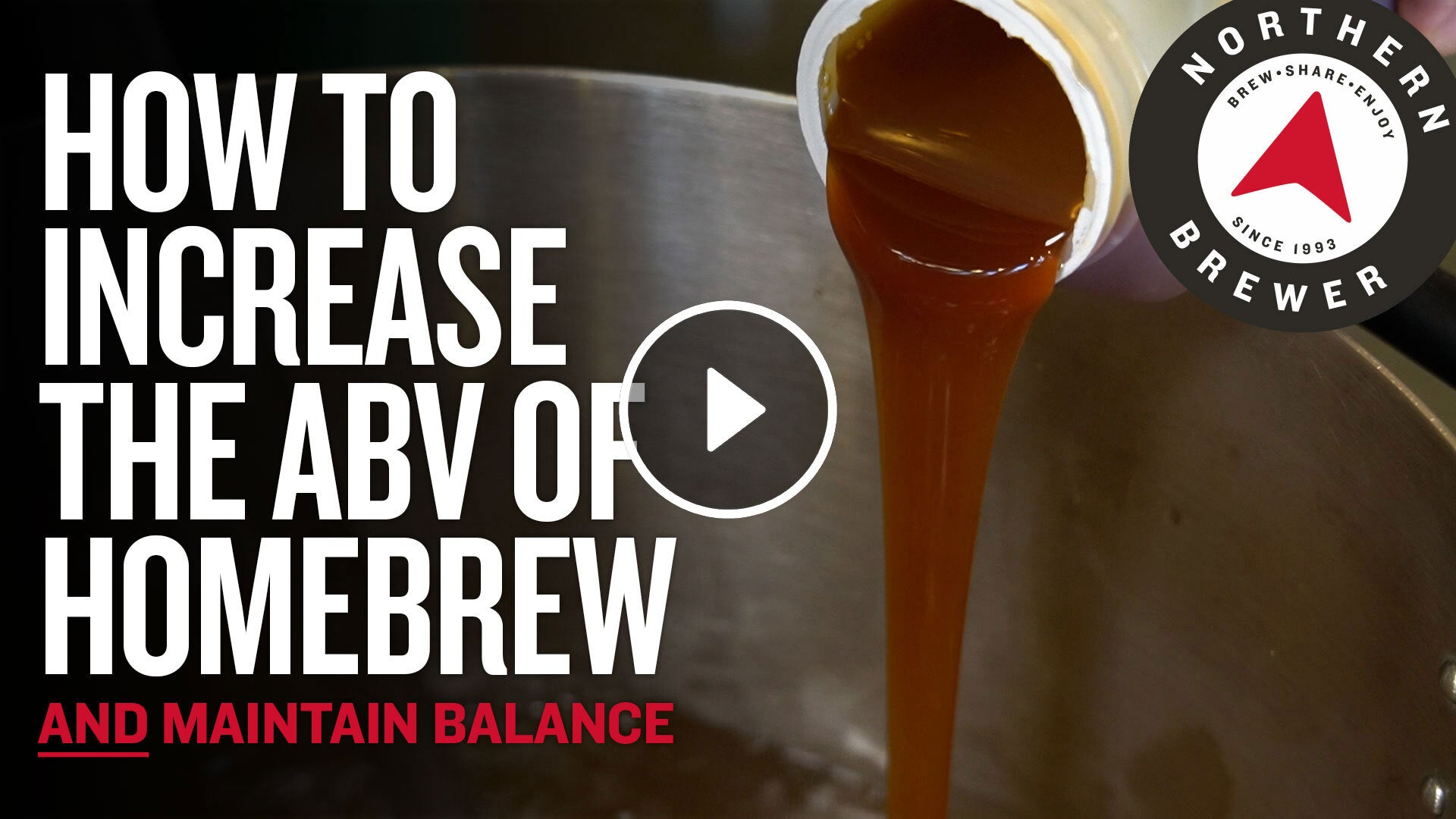# Final Gravity of Homebrew

The Final Gravity is the specific gravity measured at the completion of fermentation and represents the amount of unfermentable sugars remaining in the beer.  You can calculate the approximate alcohol by volume (abv) of the beer by subtracting the original gravity (OG) from the final gravity (FG) and multiplying it by 131: (OG-FG)*131=abv.

You can estimate the approximate finishing gravity of a beer by taking into account the attenuation rate of the yeast strain you are using.  For example if you have a yeast with a 75% attenuation rate and your original gravity is 1.050 the estimated final gravity would be about 1.012.  This is merely a estimate and can be lower or higher because there are many factors that affect the final gravity. These factors include fermentation temperature, amount of yeast pitched, the health of yeast, the amount of O2 present, mash temperature, the amount of adjuncts used, the amount of nutrients available in the wort, and the flocculation rate of the yeast strain.

For helpful tips on how to boost the ABV of your homebrew, watch the video below.